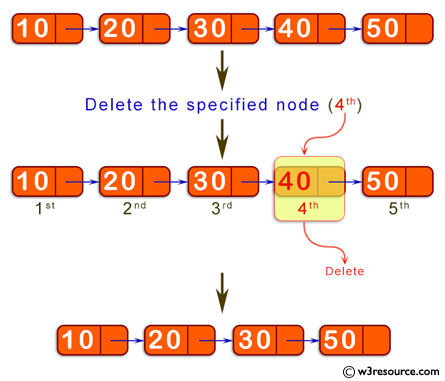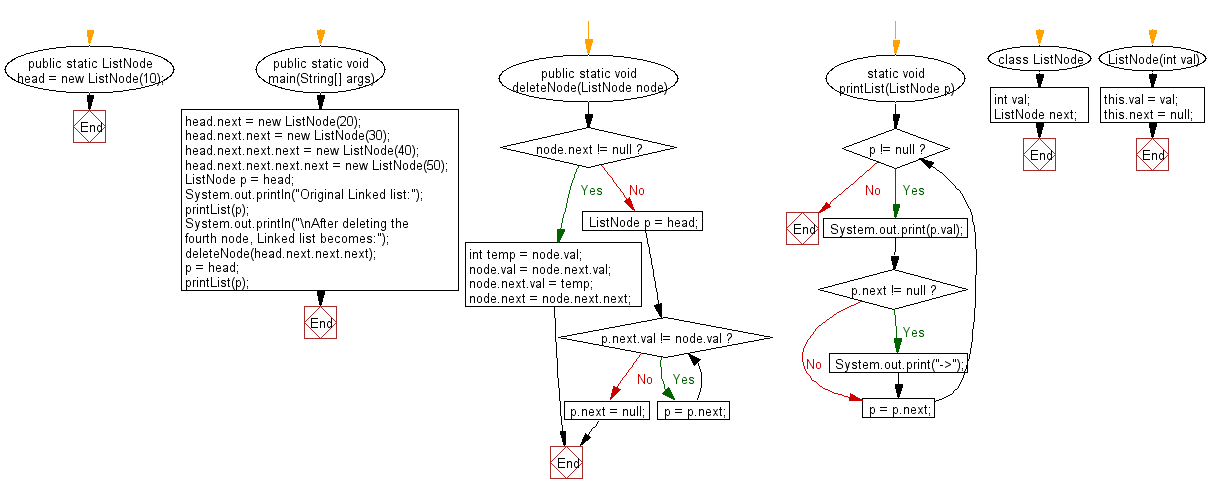﻿ Java programming exercises: Delete a specified node in the middle of a singly linked list - w3resource# Java Exercises: Delete a specified node in the middle of a singly linked list

## Java Basic: Exercise-175 with Solution

Write a Java program to delete a specified node in the middle of a singly linked list.

Delete the fourth node i.e. 40
Result: 10->20->30->50

Pictorial Presentation:Sample Solution:

Java Code:

``````import java.util.*;
import java.util.Arrays;

public class Solution {
public static ListNode head = new ListNode(10);

public static void main(String[] args) {
printList(p);
System.out.println("\nAfter deleting the fourth node, Linked list becomes:");
printList(p);
}

public static void deleteNode(ListNode node) {
if (node.next != null) {
int temp = node.val;
node.val = node.next.val;
node.next.val = temp;

node.next = node.next.next;
} else {
while (p.next.val != node.val) {
p = p.next;
}
p.next = null;
}
}

static void printList(ListNode p) {

while (p != null) {
System.out.print(p.val);
if (p.next != null) {
System.out.print("->");
}
p = p.next;
}
}
}
class ListNode {
int val;
ListNode next;

ListNode(int val) {
this.val = val;
this.next = null;
}
}
```
```

Sample Output:

```Original Linked list:
10->20->30->40->50
After deleting the fourth node, Linked list becomes:
10->20->30->50
```

Flowchart:Java Code Editor:

Company:

What is the difficulty level of this exercise?

﻿

## Java: Tips of the Day

Parsing dates:

```import java.io.*;
import java.util.*;
import java.text.*;

String s = "2001/09/23 14:39";

SimpleDateFormat formatter = new SimpleDateFormat ("yyyy/MM/dd H:mm");
Date d = formatter.parse(s, new ParsePosition(0));
```